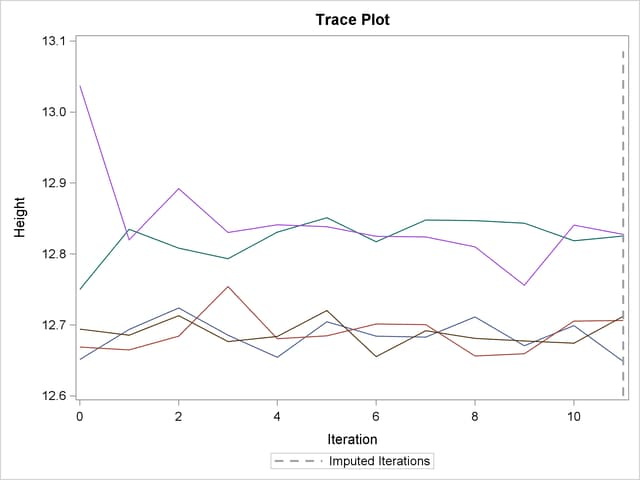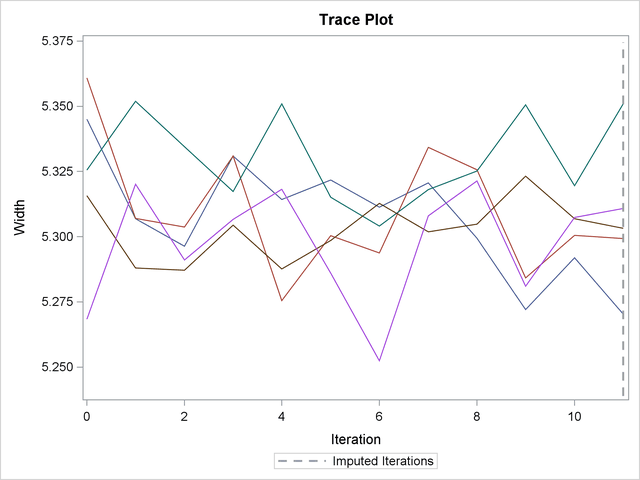## Example 56.8 FCS Method with Trace Plot

This example uses FCS methods to impute missing values in both continuous and classification variables in a data set with an arbitrary missing pattern. The following statements use a logistic regression method to impute values of the classification variable Species:

```ods graphics on;
proc mi data=Fish3 seed=1305417 out=outex8;
class Species;
fcs plots=trace
logistic(Species= Height Width Height*Width /details);
var Species Height Width;
run;
ods graphics off;
```

The "Model Information"  table in Output 56.8.1 describes the method and options used in the multiple imputation process. By default, a regression method is used to impute missing values in each continuous variable.

Output 56.8.1 Model Information
The MI Procedure

Model Information
Data Set WORK.FISH3
Method FCS
Number of Imputations 5
Number of Burn-in Iterations 10
Seed for random number generator 1305417

The "FCS Model Specification"  table in Output 56.8.2 describes methods and imputed variables in the imputation model. The procedure uses the logistic regression method to impute the variable Species, and the regression method to impute variables Height and Width.

Output 56.8.2 FCS Model Specification
FCS Model Specification
Method Imputed Variables
Regression Height Width
Logistic Regression Species

The "Missing Data Patterns" table in Output 56.8.3 lists distinct missing data patterns with corresponding frequencies and percentages. With the default ORDER=FREQ option, variables are ordered by the descending frequency counts for the missing values in the filled-in and imputation phases.

Output 56.8.3 Missing Data Patterns
Missing Data Patterns
Group Height Width Species Freq Percent Group Means
Height Width
1 X X X 40 76.92 12.627350 5.347450
2 X X . 3 5.77 11.797667 4.587667
3 X . X 1 1.92 18.037000 .
4 X . . 4 7.69 13.346750 .
5 . X . 2 3.85 . 5.135000
6 O O O 2 3.85 . .

When you use the DETAILS keyword in the LOGISTIC option, parameters estimated from the observed data and the parameters used in each imputation are displayed in the "Logistic Models for FCS Method" table in Output 56.8.4.

Output 56.8.4 FCS Logistic Regression Model for Species
Logistic Models for FCS Method
Imputed
Variable
Effect Imputation
1 2 3 4 5
Species Intercept 27.019602 27.064278 27.262198 27.214159 27.727730
Species Height 60.068695 60.007370 59.980982 59.933904 61.324682
Species Width -25.537953 -25.661405 -26.044380 -25.987921 -23.681898
Species Height*Width -5.479559 -5.839848 -6.786713 -6.691049 -2.690170

With ODS Graphics enabled, the PLOTS=TRACE option displays trace plots of means for all continuous variables by default, as shown in Output 56.8.5 and Output 56.8.6. The dashed vertical lines indicate the imputed iterations—that is, the variable values used in the imputations. The plot shows no apparent trends for the two variables.

Output 56.8.5 Trace Plot for HeightOutput 56.8.6 Trace Plot for WidthThe following statements list the first 10 observations of the data set outex8 in Output 56.8.7:

```proc print data=outex8(obs=10);
title 'First 10 Observations of the Imputed Data Set';
run;
```

Output 56.8.7 Imputed Data Set
 First 10 Observations of the Imputed Data Set

Obs _Imputation_ Species Length Height Width
1 1 Bream 30.0000 11.5200 4.02000
2 1 Bream 31.2000 12.4800 4.30600
3 1 Bream 31.1000 12.3780 4.69600
4 1 Bream 33.5000 12.7300 4.45600
5 1 Bream 23.9427 12.4440 3.35343
6 1 Bream 34.7000 13.6020 4.92700
7 1 Bream 34.5000 14.1800 5.27900
8 1 Bream 35.0000 14.8409 4.69000
9 1 Bream 35.1000 14.0050 4.84400
10 1 Bream 36.2000 14.2270 4.95900

After the completion of five imputations by default, the "Variance Information" table in Output 56.8.8 displays the between-imputation variance, within-imputation variance, and total variance for combining complete-data inferences for continuous variables. The relative increase in variance due to missingness, the fraction of missing information, and the relative efficiency for each variable are also displayed. These statistics are described in the section Combining Inferences from Multiply Imputed Data Sets.

Output 56.8.8 Variance Information
Variance Information
Variable Variance DF Relative
Increase
in Variance
Fraction
Missing
Information
Relative
Efficiency
Between Within Total
Height 0.006302 0.313539 0.321101 45.714 0.024119 0.023821 0.995258
Width 0.001343 0.017068 0.018680 39.861 0.094387 0.089626 0.982390

The "Parameter Estimates" table in Output 56.8.9 displays a 95% mean confidence interval and a t statistic with its associated p-value for each of the hypotheses requested with the default MU0=0 option.

Output 56.8.9 Parameter Estimates
Parameter Estimates
Variable Mean Std Error 95% Confidence Limits DF Minimum Maximum Mu0 t for H0:
Mean=Mu0
Pr > |t|
Height 12.744021 0.566658 11.60321 13.88484 45.714 12.648427 12.827767 0 22.49 <.0001
Width 5.303250 0.136673 5.02699 5.57951 39.861 5.256781 5.341640 0 38.80 <.0001